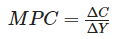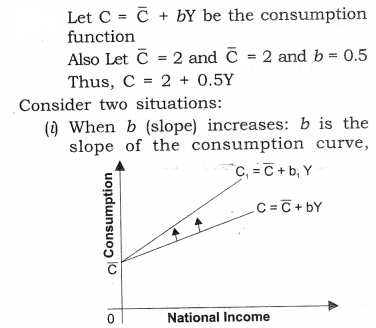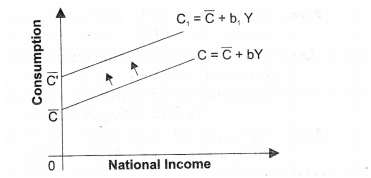• +91 9971497814
• info@interviewmaterial.com

# Chapter 5- Aggregate Demand and Its Related Concepts Interview Questions Answers

### Related Subjects

Question 1 : What is marginal propensity to consume? How is it related to marginal propensity to save?

Answer 1 : The ratio of change in consumption (C) to change in income (Y) is known as marginal propensity to consume. It indicates the proportion of additional income that is being spent on consumption.The sum of MPC and MPS is equal to one. It can be proved as under: We know: ΔY = ΔC + ΔS
Dividing both sides by AY, we get,ΔY/ΔY=ΔC/ΔY + ΔS/ΔY or 1=MPC+MPS
[ΔY/ΔY=1; ΔC/ΔY =MPC ΔS/ΔY=MPS]
MPC + MPS = 1 because total increment in income is either used for consumption or for saving.

Question 2 : What do you understand by ‘para¬metric shift of a line’? How does a line shift when its (i) slope increases and (ii) its intercept increases?when b increases from 0.5 to 0.75, then consumption curve (which is a straight line) pivots upwards. This is called a parametric shift of a graph. It is shown in figure.
(ii)When C increases: It is the autonomous part of the consumption function. It graphically gives the intercept of the consumption curve. If increases from 2 to 5, there will be parallel upward shift in the consumption curves. It is shown in figure given.Thus, when slope changes, there is parametric shift in the curve. When intercept changes, there is parallel shift in the curve.

Todays Deals### Chapter 5- Aggregate Demand and Its Related Concepts Contributorskrishan

Name:
Email:

# Latest News# 9000 interview questions in different categories